## Hexboards and Heytings

A couple of days ago, Peter Rowlett posted on The Aperiodical: Introducing hexboard – a LaTeX package for drawing games of Hex.

Hex is a strategic game with two players (Red and Blue) taking turns placing a stone of their color onto any empty space. A player wins when they successfully connect their sides together through a chain of adjacent stones.

Here’s a short game on a $5 \times 5$ board (normal play uses $11\times 11$ boards), won by Blue, drawn with the LaTeX-package hexboard.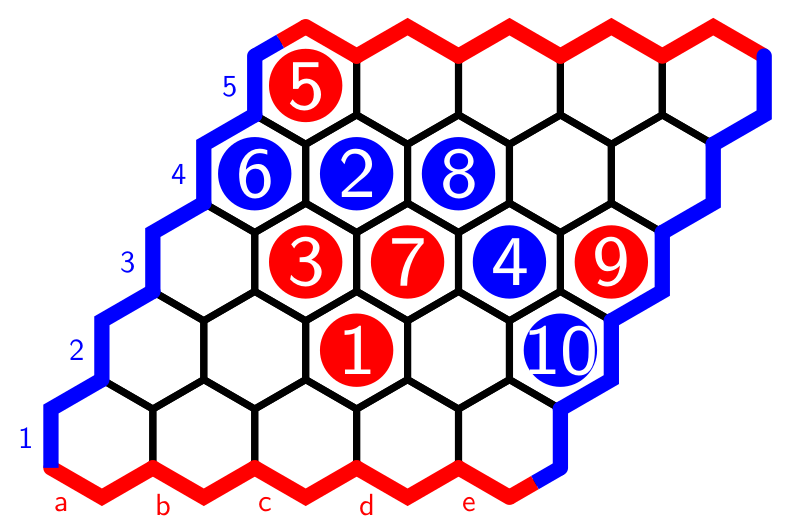As much as I like mathematical games, I want to use the versability of the hexboard-package for something entirely different: drawing finite Heyting algebras in which it is easy to visualise the logical operations.

Every full hexboard is a poset with minimal cell $0$ and maximal cell $1$ if cell-values increase if we move horizontally to the right or diagonally to the upper-right. With respect to this order, $p \vee q$ is the smallest cell bigger than both $p$ and $q$, and $p \wedge q$ is the largest cell smaller than $p$ and $q$.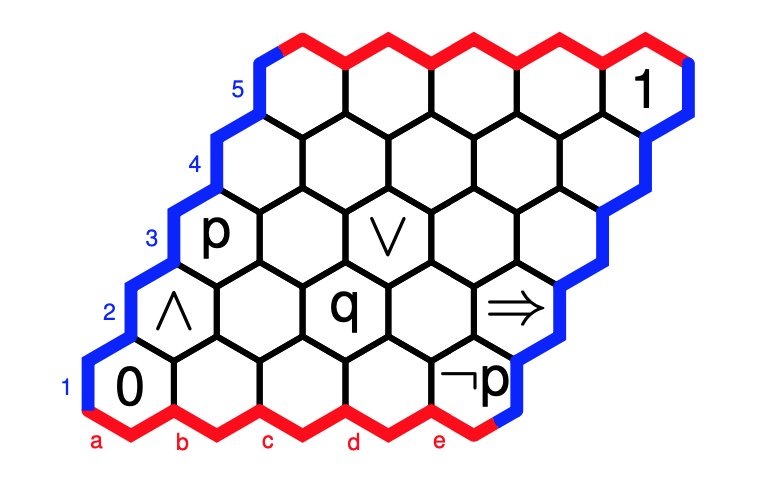The implication $p \Rightarrow q$ is the largest cell $r$ such that $r \wedge p \leq q$, and the negation $\neg p$ stands for $p \Rightarrow 0$. With these operations, the full hexboard becomes a Heyting algebra.

Now the fun part. Every filled area of the hexboard, bordered above and below by a string of strictly increasing cells from $0$ to $1$ is also a Heyting algebra, with the induced ordering, and with the logical operations defined similarly.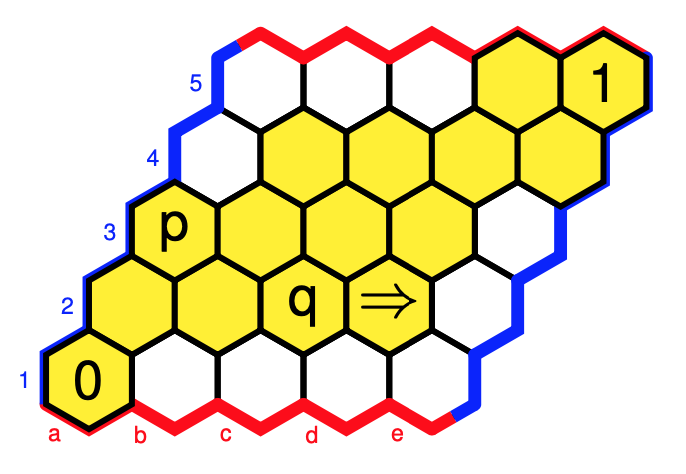Note that this mustn’t be a sub-Heyting algebra as the operations may differ. Here, we have a different value for $p \Rightarrow q$, and $\neg p$ is now $0$.

If you’re in for an innocent “Where is Wally?”-type puzzle: $W = (\neg \neg p \Rightarrow p)$.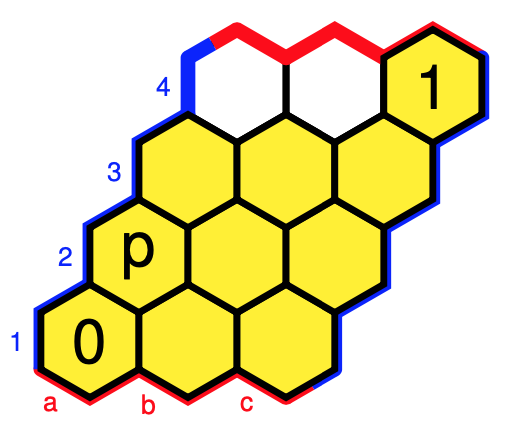Click on the image to get the solution.

The downsets in these posets can be viewed as the open sets of a finite topology, so these Heyting algebra structures come from the subobject classifier of a topos.

There are more interesting toposes with subobject classifier determined by such hex-Heyting algebras.

For example, the Topos of Triads of Thomas Noll in music theory has as its subobject classifier the hex-Heyting algebra (with cell-values as in the paper):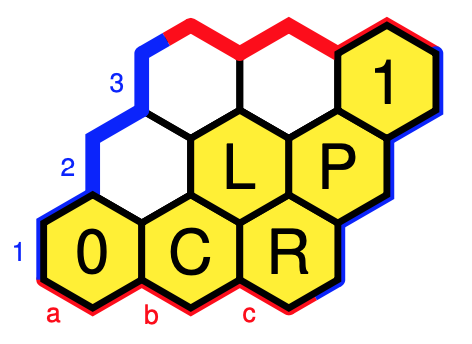Note to self: why not write a couple of posts on this topos?

Another example: the category of all directed graphs is the presheaf topos of the two object category ($V$ for vertices, and $E$ for edges) with (apart from the identities) just two morphisms $s,t : V \rightarrow E$ (for start- and end-vertex of a directed edge).

The subobject classifier $\Omega$ of this topos is determined by the two Heyting algebras $\Omega(E)$ and $\Omega(V)$ below.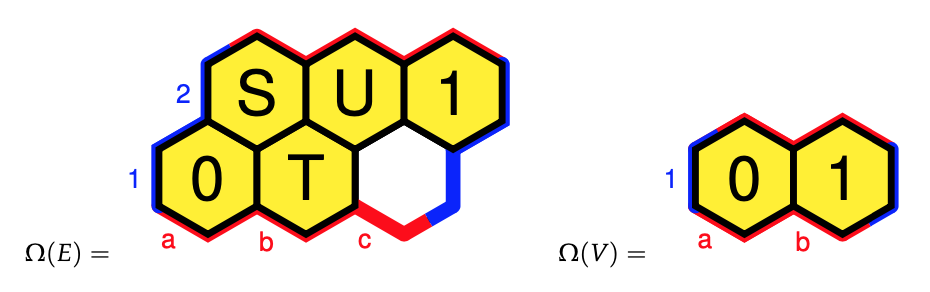These ‘hex-Heyting algebras’ are exactly what Eduardo Ochs calls ‘planar Heyting algebras’.

Eduardo has a very informative research page, containing slides and handouts of talks in which he tries to explain topos theory to “children” (using these planar Heyting algebras) including:

Perhaps now is a good time to revive my old sga4hipsters-project.

## Learners’ logic

In the Learners and Poly-post we’ve seen that learners from $A$ to $B$ correspond to set-valued representations of a directed graph $G$ and therefore form a presheaf topos.

Any topos comes with its Mitchell-Benabou language, allowing us to speak of formulas, propositions and their truth values. Two objects play a special role in this: the terminal object $\mathbf{1}$, and the subobject classifier $\mathbf{\Omega}$. It is a fun exercise to determine these special learners.

$T$ is the free rooted tree with branches sprouting from every node $n \in T_0$ for each element in $A \times B$. $C$ will be our set of colours, one for each element of $Maps(A,B) \times Maps(A \times B,A)$.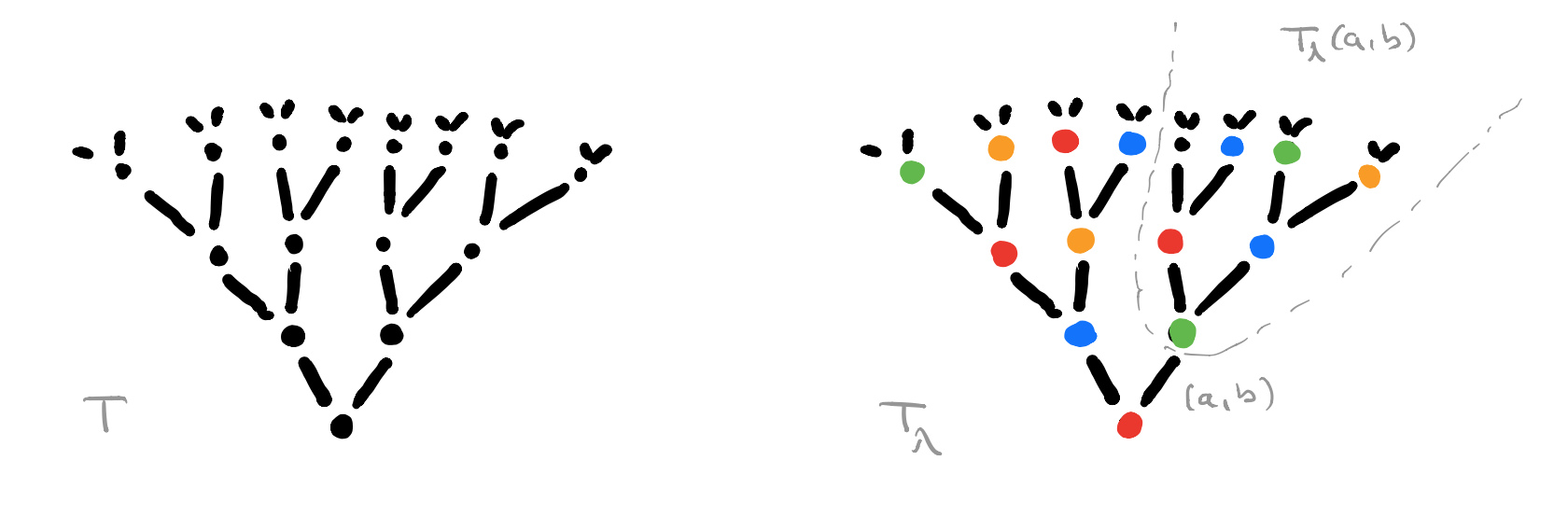For every map $\lambda : T_0 \rightarrow C$ we get a coloured rooted tree $T_{\lambda}$, and for each branch $(a,b)$ from the root we get another rooted sub-tree $T_{\lambda}(a,b)$ which is again of the form $T_{\mu}$ for a certain map $\mu : T_0 \rightarrow C$.

The directed graph $G$ has a vertex $v_{\lambda} \in V$ for each coloured rooted tree $T_{\lambda}$ and a directed edge $v_{\lambda} \rightarrow v_{\mu}$ if $T_{\mu}$ is the isomorphism class of coloured rooted trees of the subtree $T_{\lambda}(a,b)$ for some $(a,b) \in A \times B$.

There are exactly $\# A \times B$ directed edges leaving every vertex in $G$, but there may be (many) more incoming edges. We can colour each vertex $v_{\lambda}$ with the colour of the root of $T_{\lambda}$.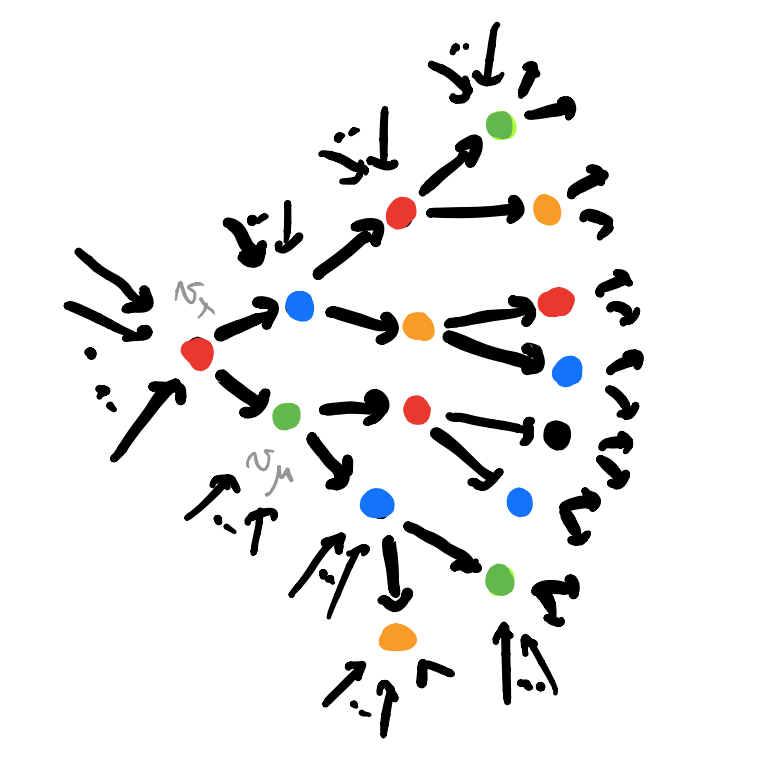The coloured directed graph $G$ depicts the learning process in a neural network, being trained to find a suitable map $A \rightarrow B$. The colour of a vertex $v_{\lambda}$ gives a map $f \in Maps(A,B)$ (and a request function). If the network now gives as output $b \in B$ for a given input $a \in A$, we can move on to the end-vertex $v_{\mu}$ of the directed edge labeled $(a,b)$ out of $v_{\lambda}$. The colour of $v_{\mu}$ gives us a new (hopefully improved) map $f_{new} \in Maps(A,B)$ (and a new request function). A new training data $(a’,b’)$ brings us to a new vertex and map, and so on.

Clearly, some parts of $G$ are more efficient to find the desired map than others, and the aim of the game is to distinguish efficient from inefficient learners. A first hint that Grothendieck topologies and their corresponding sheafifications will turn out to be important.

We’ve seen that a learner, that is a morphism $Py^P \rightarrow C y^{A \times B}$ in $\mathbf{Poly}$, assigns a set $P_{\lambda}$ to every vertex $v_{\lambda}$ (this set may be empty) and a map $P_{\lambda} \rightarrow P_{\mu}$ to every directed edge $v_{\lambda} \rightarrow v_{\mu}$ in $G$.

The terminal object $\mathbf{1}$ in this setting assigns to each vertex a singleton $\{ \ast \}$, and the obvious maps for each directed edge. In $\mathbf{Poly}$-speak, the terminal object is the morphism
$\mathbf{1}~:~V y^V \rightarrow C y^{A \times B}$
which sends each vertex $v_{\lambda} \in V$ to its colour $c \in C$, and where the backtrack map $\varphi^{\#}_{v_{\lambda}}[c]$ maps $(a,b)$ to $v_{\mu}$ if this is the end-vertex of the edge labelled $(a,b)$ out of $v_{\lambda}$. That is, $\mathbf{1}$ contains all information about the coloured directed graph $G$.

The subobject classifier $\mathbf{\Omega}$ assigns to each vertex $v_{\lambda}$ the set $\mathbf{\Omega}(v_{\lambda})$ of all subsets $S$ of directed paths in $G$, starting at $v_{\lambda}$, such that if $p \in S$ then also all prolongated paths belong to $S$. Note that the emptyset $\emptyset$ satisfies this requirement, so is an element of this vertex set. Another special element in $\mathbf{\Omega}(v_{\lambda})$ is the set $\mathbf{1}_{\lambda}$ of all oriented paths starting at $v_{\lambda}$.

$\mathbf{\Omega}(v_{\lambda})$ is an Heyting algebra with $1=\mathbf{1}_{\lambda}$, $0 = \emptyset$, partially ordered via inclusion, and logical operations $\wedge$ (intersection), $\vee$ (union), $\neg$ (with $\neg S$ the largest $S’ \in \mathbf{\Omega}(v_{\lambda})$ disjoint from $S$) and $\Rightarrow$ defined by $S \Rightarrow S’$ is the union of all $S” \in \mathbf{\Omega}(v_{\lambda})$ such that $S” \cap S \subseteq S’$.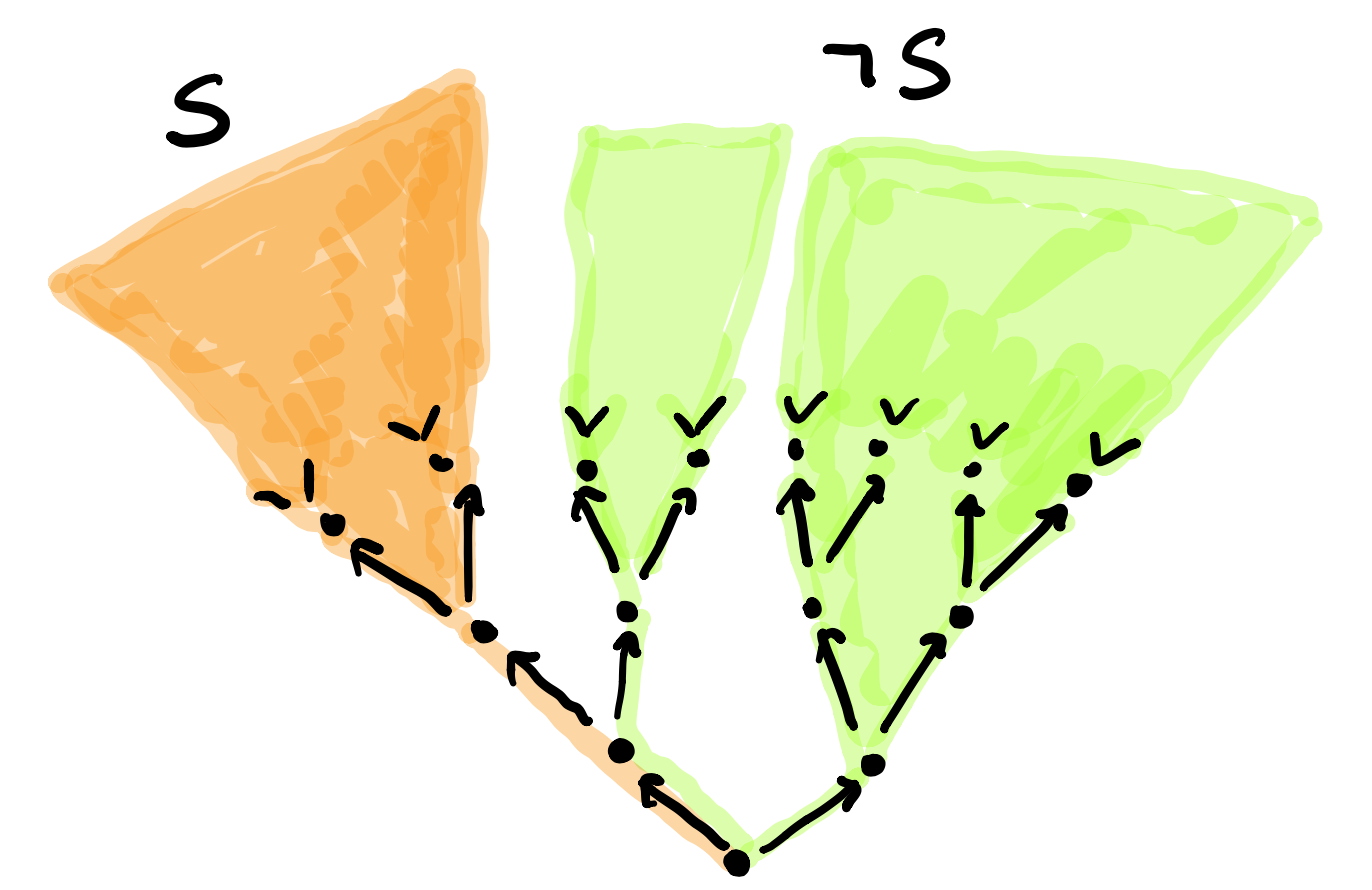$S \wedge \neg S$ is not always equal to $1$. Here, the union misses the left edge from the root. So, we will not be able to prove things by contradiction.

If $v_{\lambda} \rightarrow v_{\mu}$ is the directed edge labeled $(a,b)$, then the corresponding map $\mathbf{\Omega}(v_{\lambda}) \rightarrow \mathbf{\Omega}(v_{\mu})$ takes an $S \in \mathbf{\Omega}(v_{\lambda})$, drops all paths which do not pass through $v_{\mu}$ and removes from those who do the initial edge $(a,b)$. If no paths in $S$ pass through $v_{\mu}$ then $S$ is mapped to $\emptyset \in \mathbf{\Omega}(v_{\mu})$.

If $\Omega = \bigsqcup_{\lambda} \mathbf{\Omega}(v_{\lambda})$ then the subobject classifier is the morphism in $\mathbf{Poly}$
$\mathbf{\Omega}~:~\Omega y^{\Omega} \rightarrow C y^{A \times B}$
sending a path starting in $v_{\lambda}$ to the colour of $v_{\lambda}$ and the backtrack map of $(a,b)$ the image of the path under the map $\mathbf{\Omega}(v_{\lambda}) \rightarrow \mathbf{\Omega}(v_{\mu})$.

Ok, let’s define the Learner’s Mitchell-Benabou language.

We’ll view a learner $Py^P \rightarrow C y^{A \times B}$ as a set-valued representation $P$ of the directed graph $G$ with vertex set $P_{\lambda}$ placed at vertex $v_{\lambda}$.

A formula $\phi(p)$ of the language with a free variable $p$ is a morphism (of representations of $G$) from a learner $P$ to the subobject classifier
$\phi~:~P \rightarrow \mathbf{\Omega}$
Such a morphism determines a sub-representation of $P$ which we can denote $\{ p | \phi(p) \}$ with vertex sets
$\{ p | \phi(p) \}_{\lambda} = \{ p \in P_{\lambda}~|~\phi(v_{\lambda})(p) = \mathbf{1}_{\lambda} \}$

On formulas we can apply logical connectives to get more formulas. For example, the formula $\phi(p) \Rightarrow \psi(q)$ is the composition
$P \times Q \rightarrow^{\phi \times \psi} \mathbf{\Omega} \times \mathbf{\Omega} \rightarrow^{\Rightarrow} \mathbf{\Omega}$

By quantifying all free variables we get a formula without free variables, and those correspond to morphisms $\mathbf{1} \rightarrow \mathbf{\Omega}$, that is, to sub-representations of the terminal object $\mathbf{1}$.

For example, if $\phi(p)$ is the formula with free variable $p$ corresponding to the morphism $\phi : P \rightarrow \mathbf{\Omega}$, then we have
$\forall p : \phi(p) = \{ v_{\lambda} \in V~|~\{ p | \phi(p) \}_{\lambda} = P_{\lambda} \}$
and
$\exists p : \phi (p) = \{ v_{\lambda} \in V~|~\{ p | \phi(p) \}_{\lambda} \not= \emptyset \}$

Sub-representations of $\mathbf{1}$ again form a Heyting-algebra in the obvious way, so we can assign a “truth-value” to a formula without free variables as that sub-object of $\mathbf{1}$.

There’s a lot more to say, so perhaps this will be continued.

## Yet more topos news

Every topos has its own internal language, the so called Mitchell-Bénabou language, allowing us to speak about formulas and their truth values.

Sadly, Jean Bénabou died last week.

Here’s a nice interview with Bénabou (in French) on category theory, Grothendieck, logic, and a rant on plagiarism among topos theorists (starting at 1:00:16).

Yesterday, France Culture’s ‘La methode scientifique’ hosted Alain Connes, Laurent Lafforgue and Olivia Caramello in a special programme Grothendieck: la moisson (Grothendieck, the harvest), dedicated to the recent publication of ‘Récoltes et Semailles’.

An interesting item is ‘le reportage du jour’ by Céline Loozen in which she manages to have a look at the 60.000 pages of Grothendieck’s Lasserre notes, stocked in the cellars of the Librairie Alain Brieux, and talks to Jean-Bernard Gillot who is commissioned by Grothendieck’s son to appraise the work (starts at 36:40).

Perhaps the publication of ‘Récoltes et Semailles’ is part of a deal with the family to make these notes available, at last.

Towards the end of the programme Connes, Caramello and Lafforgue lament that topos theory is still not taken seriously by the mathematical community at large, whereas it is welcomed warmly by the engineers of Huawei.

In more topos news, I learn from the blog of Olivia Caramello, that Laurent Lafforgue is going to give an online course on toposes as ‘bridges’ at the University of Warwick, the first talk starts today at 14hrs London time.

## The hype cycle of an idea

These three ideas (re)surfaced over the last two decades, claiming to have potential applications to major open problems:

• (2000) $\mathbb{F}_1$-geometry tries to view $\mathbf{Spec}(\mathbb{Z})$ as a curve over the field with one element, and mimic Weil’s proof of RH for curves over finite fields to prove the Riemann hypothesis.
• (2012) IUTT, for Inter Universal Teichmuller Theory, the machinery behind Mochizuki’s claimed proof of the ABC-conjecture.
• (2014) topos theory : Connes and Consani redirected their RH-attack using arithmetic sites, while Lafforgue advocated the use of Caramello’s bridges for unification, in particular the Langlands programme.

It is difficult to voice an opinion about the (presumed) current state of such projects without being accused of being either a believer or a skeptic, resorting to group-think or being overly critical.

We lack the vocabulary to talk about the different phases a mathematical idea might be in.

Such a vocabulary exists in (information) technology, the five phases of the Gartner hype cycle to represent the maturity, adoption, and social application of a certain technology :

1. Technology Trigger
2. Peak of Inflated Expectations
3. Trough of Disillusionment
4. Slope of Enlightenment
5. Plateau of ProductivityThis model can then be used to gauge in which phase several emerging technologies are, and to estimate the time it will take them to reach the stable plateau of productivity. Here’s Gartner’s recent Hype Cycle for emerging Artificial Intelligence technologies.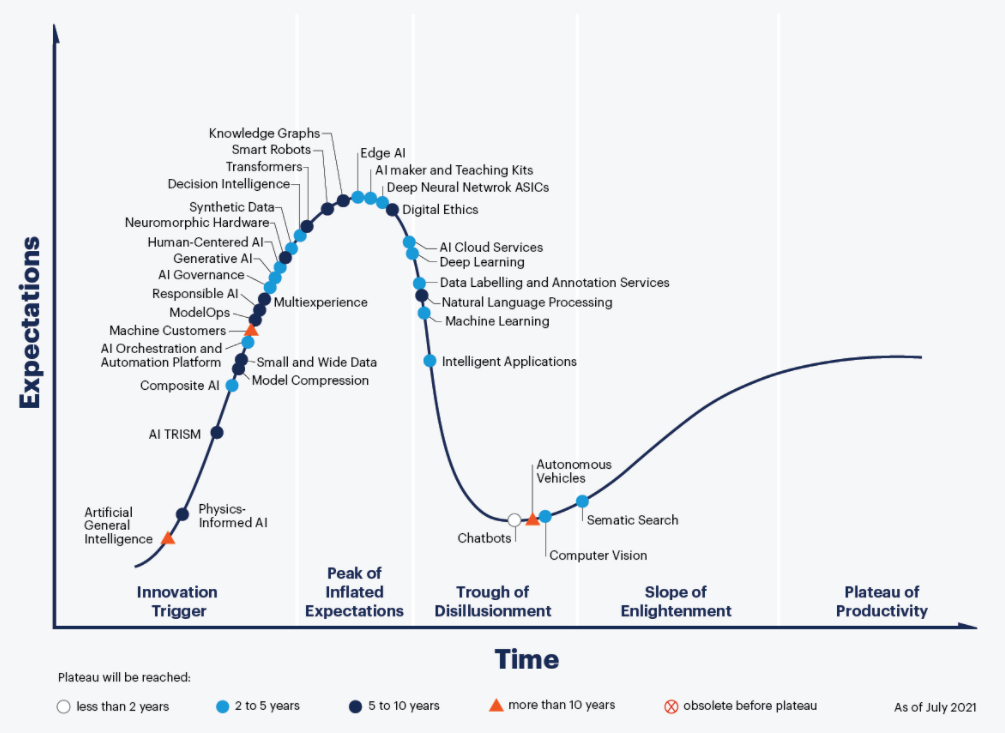Picture from Gartner Hype Cycle for AI 2021

What might these phases be in the hype cycle of a mathematical idea?

1. Technology Trigger: a new idea or analogy is dreamed up, marketed to be the new approach to that problem. A small group of enthusiasts embraces the idea, and tries to supply proper definitions and the very first results.
2. Peak of Inflated Expectations: the idea spreads via talks, blogposts, mathoverflow and twitter, and now has enough visibility to justify the first conferences devoted to it. However, all this activity does not result in major breakthroughs and doubt creeps in.
3. Trough of Disillusionment: the project ran out of steam. It becomes clear that existing theories will not lead to a solution of the motivating problem. Attempts by key people to keep the idea alive (by lengthy papers, regular meetings or seminars) no longer attract new people to the field.
4. Slope of Enlightenment: the optimistic scenario. One abandons the original aim, ditches the myriad of theories leading nowhere, regroups and focusses on the better ideas the project delivered.

A negative scenario is equally possible. Apart for a few die-hards the idea is abandoned, and on its way to the graveyard of forgotten ideas.

5. Plateau of Productivity: the polished surviving theory has applications in other branches and becomes a solid tool in mathematics.

It would be fun so see more knowledgable people draw such a hype cycle graph for recent trends in mathematics.

Here’s my own (feeble) attempt to gauge where the three ideas mentioned at the start are in their cycles, and here’s why:

• IUTT: recent work of Kirti Joshi, for example this, and this, and that, draws from IUTT while using conventional language and not making exaggerated claims.
• $\mathbb{F}_1$: the preliminary programme of their seminar shows little evidence the $\mathbb{F}_1$-community learned from the past 20 years.
• Topos: Developing more general theory is not the way ahead, but concrete examples may carry surprises, even though Gabriel’s topos will remain elusive.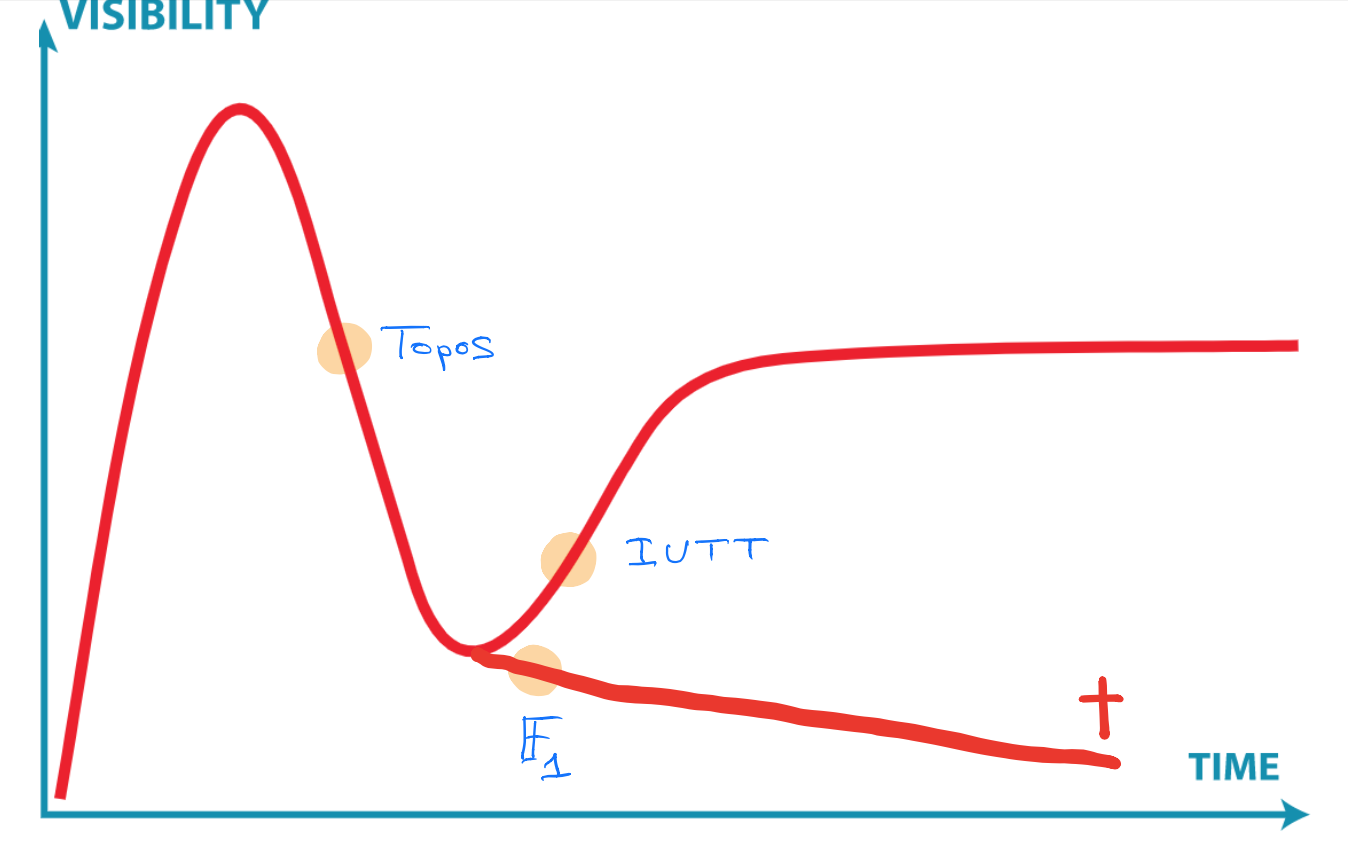Clearly, you don’t agree, and that’s fine. We now have a common terminology, and you can point me to results or events I must have missed, forcing me to redraw my graph.

## Grothendieck stuff

January 13th, Gallimard published Grothendieck’s text Recoltes et Semailles in a fancy box containing two books.Here’s a G-translation of Gallimard’s blurb:

“Considered the mathematical genius of the second half of the 20th century, Alexandre Grothendieck is the author of Récoltes et semailles, a kind of “monster” of more than a thousand pages, according to his own words. The mythical typescript, which opens with a sharp criticism of the ethics of mathematicians, will take the reader into the intimate territories of a spiritual experience after having initiated him into radical ecology.

In this literary braid, several stories intertwine, “a journey to discover a past; a meditation on existence; a picture of the mores of a milieu and an era (or the picture of the insidious and implacable shift from one era to another…); an investigation (almost police at times, and at others bordering on the swashbuckling novel in the depths of the mathematical megapolis…); a vast mathematical digression (which will sow more than one…); […] a diary ; a psychology of discovery and creation; an indictment (ruthless, as it should be…), even a settling of accounts in “the beautiful mathematical world” (and without giving gifts…)”.”

All literary events, great or small, are cause for the French to fill a radio show.

January 21st, ‘Le grand entretien’ on France Inter invited Cedric Villani and Jean-Pierre Bourguignon to talk about Grothendieck’s influence on mathematics (h/t Isar Stubbe).

The embedded YouTube above starts at 12:06, when Bourguignon describes Grothendieck’s main achievements.

Clearly, he starts off with the notion of schemes which, he says, proved to be decisive in the further development of algebraic geometry. Five years ago, I guess he would have continued mentioning FLT and other striking results, impossible to prove without scheme theory.

Now, he goes on saying that Grothendieck laid the basis of topos theory (“to define it, I would need not one minute and a half but a year and a half”), which is only now showing its first applications.

Grothendieck, Bourguignon goes on, was the first to envision the true potential of this theory, which we should take very seriously according to people like Lafforgue and Connes, and which will have applications in fields far from algebraic geometry.

Topos20 is spreading rapidly among French mathematicians. We’ll have to await further results before Topos20 will become a pandemic.

Another interesting fragment starts at 16:19 and concerns Grothendieck’s gribouillis, the 50.000 pages of scribblings found in Lasserre after his death.

Bourguignon had the opportunity to see them some time ago, and when asked to describe them he tells they are in ‘caisses’ stacked in a ‘libraire’.

Here’s a picture of these crates taken by Leila Schneps in Lasserre around the time of Grothendieck’s funeral.If you want to know what’s in these notes, and how they ended up at that place in Paris, you might want to read this and that post.

If Bourguignon had to consult these notes at the Librairie Alain Brieux, it seems that there is no progress in the negotiations with Grothendieck’s children to make them public, or at least accessible.